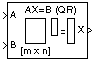# QR Solver

Find minimum-norm-residual solution to AX=B

## Library

Math Functions / Matrices and Linear Algebra / Linear System Solvers

`dspsolvers`

•## Description

The QR Solver block solves the linear system AX=B, which can be overdetermined, underdetermined, or exactly determined. The system is solved by applying QR factorization to the M-by-N matrix, A, at the `A` port. The input to the `B` port is the right side M-by-L matrix, B. The block treats length-M unoriented vector input as an M-by-1 matrix.

The output at the `x` port is the N-by-L matrix, X. X is chosen to minimize the sum of the squares of the elements of B-AX. When B is a vector, this solution minimizes the vector 2-norm of the residual (B-AX is the residual). When B is a matrix, this solution minimizes the matrix Frobenius norm of the residual. In this case, the columns of X are the solutions to the L corresponding systems AXk=Bk, where Bk is the kth column of B, and Xk is the kth column of X.

X is known as the minimum-norm-residual solution to AX=B. The minimum-norm-residual solution is unique for overdetermined and exactly determined linear systems, but it is not unique for underdetermined linear systems. Thus when the QR Solver is applied to an underdetermined system, the output X is chosen such that the number of nonzero entries in X is minimized.

## Parameters

expand all

• `Interpreted execution`

Simulate model using the MATLAB® interpreter. This option shortens startup time and has faster simulation speed compared to ```Code generation```.

• `Code generation`

Simulate model using generated C code. The first time you run a simulation, Simulink® generates C code for the block. The C code is reused for subsequent simulations, as long as the model does not change. This option requires additional startup time but provides faster subsequent simulations.

## Algorithm

QR factorization factors a column-permuted variant (Ae) of the M-by-N input matrix A as

Ae = QR

where Q is a M-by-min(M,N) unitary matrix, and R is a min(M,N)-by-N upper-triangular matrix.

The factored matrix is substituted for Ae in

AeX = Be

and

QRX = Be

is solved for X by noting that Q-1 = Q* and substituting Y = Q*Be. This requires computing a matrix multiplication for Y and solving a triangular system for X.

RX = Y

## Supported Data Types

• Double-precision floating point

• Single-precision floating point

## Extended Capabilities

### Topics

Introduced before R2006a

Watch now